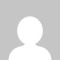Knowledgebase
KnowledgebaseNumbers - fexp, ffix, fnum, ffixr, fnumr, fcur functions. Posted by - NA - on 11 March 2005 10:52 PM Numeric values may be formatted with exponent, fixed, numeric or currency format. For this, use the functions fexp, ffix, ffixr, fnum, fnumr or fcur in report templates. For example: \fnum(a:field1)\, \fcur(a:field2,4)\, \fexp(a:field3,10:2)\   General format for all format functions is \func(value[,[precision:]decimals])\   Where func is the format function, value is a data field or variable.   The precision parameter should be 7 or less for values of type Single, 15 or less for values of type Double, and 18 or less for values of type Extended. The precision parameter may be omitted. By default precision=18.   The meaning of the decimals parameter depends on the particular function used. This parameter also may be omitted. By default decimals=2 for fexp, ffix, fnum functions. Decimals=value of global variable CurrencyDecimals (unit SysUtils) when used with fcur.   fexp(name[,[precision:]decimals]) Scientific format. The value is converted to a string of the form "-d.ddd...E+dddd". The resulting string starts with a minus sign if the number is negative, and one digit always precedes the decimal point. The total number of digits in the resulting string (including the one before the decimal point) is given by the Precision parameter. The "E" exponent character in the resulting string is always followed by a plus or minus sign and up to four digits. The Decimals parameter specifies the minimum number of digits in the exponent (between 0 and 4).   ffix(name[,[precision:]decimals]) Fixed point format. The value is converted to a string of the form "-ddd.ddd...". The resulting string starts with a minus sign if the number is negative, and at least one digit always precedes the decimal point. The number of digits after the decimal point is given by the Decimals parameter--it must be between 0 and 18. If the number of digits to the left of the decimal point is greater than the specified precision, the resulting value will use scientific format.   ffixr(name[,[precision:]decimals]) The same as ffix function, except that DecimalRSeparator property will be used instead of default decimal separator char. DecimalRTerminator char will be at the end of integer values.   fnum(name[,[precision:]decimals]) Number format. The value is converted to a string of the form "-d,ddd,ddd.ddd...". The fNum function corresponds to the fFix function, except that the resulting string contains thousand separators.   fnumr(name[,[precision:]decimals]) The same as fnum function, except that DecimalRSeparator property will be used instead of default decimal separator char. DecimalRTerminator char will be at the end of integer values.   fcur(name[,[precision:]decimals]) Currency format. The value is converted to a string that represents a currency amount. The conversion is controlled by the CurrencyString, CurrencyFormat, NegCurrFormat, ThousandSeparator, and DecimalSeparator global variables, all of which are initialized from the Currency Format in the International section of the Windows Control Panels. The number of digits after the decimal point is given by the Decimals parameter--it must be between 0 and 18.(267 vote(s))HelpfulNot helpful